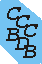Computational Chemistry Comparison and Benchmark DataBase Release 19 (April 2018) Standard Reference Database 101 National Institute of Standards and Technology Home All data for one species Geometry Experimental Calculated Comparisons Bad Calculations Tutorials and Explanations Vibrations Experimental Calculated Scale factors Reactions Entropies Ions List Ions Energy Electron Affinity Proton Affinity Ionization changes point group Experimental One molecule all properties One property a few molecules Geometry Vibrations Energy Electrostatics Reference Data Calculated Energy Optimized Reaction Internal Rotation Orbital Nuclear repulsion energy Correlation Ion Excited State Basis Set Extrapolation Geometry Vibrations Frequencies Zero point energy (ZPE) Scale Factors Bad Calculations Electrostatics Charges Dipole Quadrupole Polarizability Spin Entropy and Heat Capacity Reaction Lookup by property Comparisons Geometry Vibrations Energy Entropy Electrostatics Ion Resources Info on Results Calculations Done Basis functions used I/O files Glossary Conversion Forms Links NIST Links External links Thermochemistry Tutorials Vibrations Entropy Energy Electrostatics Geometry Cost Bad Calculations FAQ Help Units Choose Units Explanations Credits Just show me Summary Using List Recent molecules Molecules Geometry Vibrations Energy Similar molecules Ions, Dipoles, etc. Index of CCCBDB Feedback You are here: Comparisons > Vibrations > Vibrations

# Compare vibrational frequencies in CCCBDB for ClONO (chlorine nitrite)

### BLYP/6-31G

17 05 22 16 49
Frequency in cm-1
Calculated   Experimental
Mode Number Symmetry Frequency diff Symmetry Frequency Comment
1 A' 1449 -266 A' 1715
2 A' 874 16 A' 858
3 A' 689 45 A' 644
4 A' 353 -53 A' 406
5 A' 138 -132 A' 270
6 A" 441
The calculated vibrational frequencies were scaled by 0.9924

See section Calculated; Vibrations; Scale Factors; Set scaling factors to change the scale factors used here.
See section Calculated; Vibrations; Scale Factors; Calculate a scale factor to determine the least squares best scaling factor.
See section Calculated; Vibrations; Scale Factors; Scale factor uncertainty for information on where the above scaling factor is from.